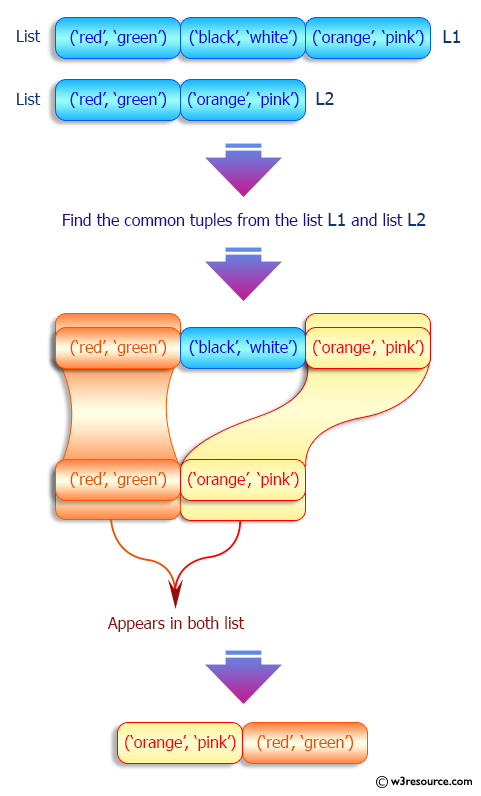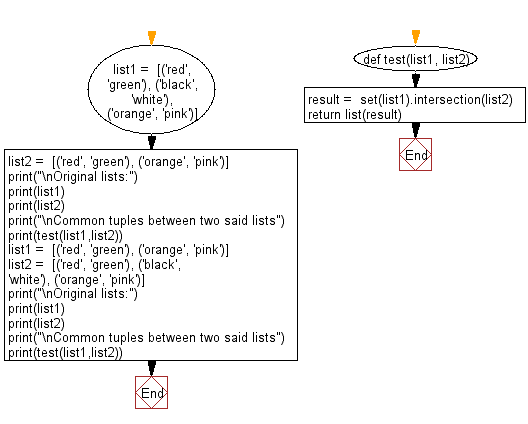﻿ Python: Common tuples between two given lists - w3resource# Python: Common tuples between two given lists

## Python List: Exercise - 207 with Solution

Write a Python program to find the common tuples between two given lists.

Sample Solution:

Python Code:

``````def test(list1, list2):
result =  set(list1).intersection(list2)
return list(result)
list1 =  [('red', 'green'), ('black', 'white'), ('orange', 'pink')]
list2 =  [('red', 'green'), ('orange', 'pink')]
print("\nOriginal lists:")
print(list1)
print(list2)
print("\nCommon tuples between two said lists")
print(test(list1,list2))
list1 =  [('red', 'green'), ('orange', 'pink')]
list2 =  [('red', 'green'), ('black', 'white'), ('orange', 'pink')]
print("\nOriginal lists:")
print(list1)
print(list2)
print("\nCommon tuples between two said lists")
print(test(list1,list2))
```
```

Sample Output:

```Original lists:
[('red', 'green'), ('black', 'white'), ('orange', 'pink')]
[('red', 'green'), ('orange', 'pink')]

Common tuples between two said lists
[('orange', 'pink'), ('red', 'green')]

Original lists:
[('red', 'green'), ('orange', 'pink')]
[('red', 'green'), ('black', 'white'), ('orange', 'pink')]

Common tuples between two said lists
[('orange', 'pink'), ('red', 'green')]
```

Pictorial Presentation:Flowchart:## Visualize Python code execution:

The following tool visualize what the computer is doing step-by-step as it executes the said program:

Python Code Editor:

Have another way to solve this solution? Contribute your code (and comments) through Disqus.

What is the difficulty level of this exercise?

Test your Python skills with w3resource's quiz

﻿

## Python: Tips of the Day

Floor Division:

When we speak of division we normally mean (/) float division operator, this will give a precise result in float format with decimals.

For a rounded integer result there is (//) floor division operator in Python. Floor division will only give integer results that are round numbers.

```print(1000 // 300)
print(1000 / 300)```

Output:

```3
3.3333333333333335```